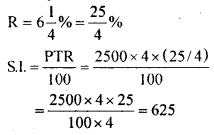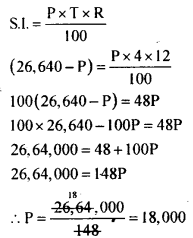# KSEEB Solutions for Class 8 Maths Chapter 9 Commercial Arithmetic Ex 9.5

Students can Download Maths Chapter 9 Commercial Arithmetic Ex 9.5 Questions and Answers, Notes Pdf, KSEEB Solutions for Class 8 Maths helps you to revise the complete Karnataka State Board Syllabus and score more marks in your examinations.

## Karnataka Board Class 8 Maths Chapter 9 Commercial Arithmetic Ex 9.5

Question 1.
Find the simple interest on Rs. 2500 for 4 years at 6 $$\frac { 1 }{ 4 }$$ % per annum.
P = Rs. 2500
T = 4 yearsSimple interest is Rs. 625.

Question 2.
Find the simple interest on Rs. 3500 at the rate of 214% per annum for 165 days.
P= Rs. 3500Simple interest = Rs. 39.55.

Question 3.
In what period will Rs. 5200 amounts to Rs. 7,384 at 12% per annum simple interest?
P = Rs. 5200
Amount = Rs. 7384
R = 12%
T = ?
Simple interest = Amount – principal = 7384 – 5200 = Rs. 2184If amounts to Rs. 7,384 in 3.5 years

Question 4.
Ramya borrowed a loan from a bank for buying a computer. After 4 years she paid Rs. 26,640 and settled the account. If the rate of interest is 12% per annum what was the sum she borrowed?
T = 4 years
R = 12%
After 4 years she paid Rs. 26,640.
This is nothing but amount.
Let the principal borrowed be R Amount = principal + S.I.
∴ Simple interest = Amount – principal S.I. = 26,640 – PThe principal borrowed – Rs. 18,000

Question 5.
A sum of money triples itself in 8 years. Find the rate of interest.